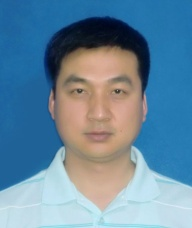Globally optimal sequential and distributed fusion state estimation for multi-sensor systems with cross-correlated noises

Significance

For efficient signal processing and design of controller systems, state estimation, such as the application of Kalman filtering theory, has been seen to be of crucial significance. In particular, the aforementioned theory has been proven to be among the most important theories owing to its wide applicability in target detection and tracking, industrial monitoring, signal processing-among other applications. Most studies on Kalman filtering are done under the assumption that the process and measurement noises are independent or uncorrelated. However, this assumption is hard to be guaranteed in many practical applications. As such, estimation problems with correlated noises have gained considerable attention in recent years.

This has resulted in the application of various distribution fusion estimation algorithms, despite their lower estimation accuracy when compared to centralized ones. Unfortunately, a thorough analysis of available text shows that the estimators for measurement noises are missing in their sequential fusion algorithm, which results in a suboptimal estimate.

In a recent publication, Heilongjiang University scientists: Dr. Shuli Sun and Dr. Honglei Lin from the School of Electrical Engineering presented a study where, the globally optimal sequential and distributed fusion estimation algorithms in the linear minimum variance (LMV) sense were developed for multi-sensor systems with correlated noises. In their approach, the measurement noises were auto- and cross-correlated with each other at the same instant and cross correlated with the system noise at the previous instant. This approach was different from other approaches in that the process and measurement noises were assumed to be independent, and correlated noises were considered. Their work is currently published in the research journal, Automatica.

To begin with, a globally optimal sequential fusion filter was proposed by considering the estimators for measurement noises. Next, the equivalence on estimation accuracy of the proposed sequential fusion filter and centralized fusion filter was proven. The authors reported that the proposed globally optimal sequential fusion filter in the LMV sense could provide real-time estimates by immediate processing data according to arriving orders of the measurements of different sensors. A comparison with the centralized fusion filter showed that the proposed sequential fusion algorithm had the advantage of reduced computational cost coupled with the same estimation accuracy. In summary, a globally optimal sequential fusion filter in the LMV sense was demonstrated by using estimators for measurement noises.

Besides, a globally optimal distributed fusion filter with feedback was also presented by fusing the prior fusion predictor and local filters. It also portrayed the same estimation accuracy as the centralized fusion filter under a general condition. Even better, a comparison with the suboptimal distributed fusion filter by matrix-weighted fusion of local filters, the proposed distributed fusion filter showed better estimation accuracy and the same computational order of magnitude. Moreover, it has a potential application in distributed estimation and consensus estimation over sensor networks or multi-agent systems.

Then, the two researchers analyzed the stability and steady-state properties of the proposed sequential and distributed fusion filtering algorithms, following which a sufficient condition for the existence of steady-state fusion filters was given. Altogether, simulation results for a target tracking system confirmed further the effectiveness of the proposed algorithms.Honglei Lin was born in Heilongjiang, China in 1986. She received the B.S. degree in department of mathematics from Mudanjiang Normal University, China, in 2010, M.E. degree in School of Mathematics science from Heilongjiang University, China, in 2013, and Ph.D. degree in School of Electrical Engineering from Heilongjiang University, China, in 2018, respectively. She is currently a Lecturer with the School of Electrical Engineering of Heilongjiang University. Her main research interests are state estimation and information fusion filtering.